Contact@EnergyStorageMedia.com

# Packed bed thermal energy storage: A simplified experimentally validated model

Thermal energy storage in packed beds is receiving increased attention as a necessary component for efficient implementation of concentrated solar power plants. A simplified, one-equation thermal model for the behavior of a packed bed is presented for a-alumina as solid storage material and air as the heat transfer fluid. The model successfully predicts the thermocline behavior over time. Two flow rates during storage are presented for alumina in a cylindrical packed bed. Temperature-dependent thermophysical properties are utilized to accurately model the systems. An additional study of air and alumina at high temperature (700 C) is presented to further highlight the importance of variable thermophysical properties in real models. Explicit consideration is given to explain situations where the modeling approach is valid based on a Biot number analysis and the thermal capacities of the solid and fluid.

## Introduction

Research in sustainable energy sources continues in order to address concerns over climate change, pollution, and nonrenewable sources. Concentrating solar power (CSP) plants are emerging as one such sustainable energy option that can generate electricity from solar energy. Systems such as solar power towers, parabolic trough collectors, and linear Fresnel reflectors concentrate solar energy by reflecting sunlight to a receiver. At the receiver, a ‘thermal energy carrier’ is heated, which is then utilized to generate power. This technology is particularly well suited to areas with high solar irradiation fluxes. However, solar energy availability is variable, such as from night to day or summer to winter, and the levelized cost of electricity (LCOE) is high. Thermal energy storage (TES) can offset variability and reduce costs.

However, storage and recovery of thermal energy must be done efficiently to achieve high capacity factors and low LCOE. As described in the review of Kuravi et al., TES technologies must meet several requirements: high energy density, good heat transfer between the heat transfer fluid (HTF) and solid storage media, stability (mechanical and chemical) of the storage medium, low thermal losses, low cost, and reversibility through many charging and discharging cycles.

A comparison of thermal energy storage designs is given here. TES can be done with sensible heat storage systems (heating a solid material) or latent heat storage (energy associated with phase change from solid to liquid). The present study explores sensible thermal energy storage; reviews of phase change based systems are provided here and here. The solid storage arrangement studied here is to store the heat in a packed bed, which is considered an emerging technology to boost total system efficiency. Charging the bed is achieved by flowing fluid, heated by solar radiation, through the packed bed to heat the storage material. To recover the stored energy from the bed (discharge), the flow direction is reversed and low temperature fluid enters the already heated bed. The exiting fluid is at a higher temperature and can then be used in a power cycle.

In packed bed systems like these, experimental and modeling studies have examined the effects of parameters such as void fraction, flow rate variations, wall thermal losses, particle size, packing material, and fluid inlet temperature. For packed beds to be efficient in thermal cycling, they must maintain a high degree of thermal stratification, which is affected by the aforementioned system parameters.

Higher exergy (a measure of useful work in the system) is recovered when little mixing of the hot and cold zones in the storage tank occurs, making the control and shape of the thermal front important ; this is readily appreciated when one considers that the maximum thermal efficiency in a Carnot cycle increases with the highest temperature. In addition to the thermal effects in the bed, the fluid flow conditions must be considered to minimize the pressure drop, which can add to exergy destruction . A low void fraction in the bed will lead to a smaller storage vessel for a given amount of energy to be stored, but the pressure drop is increased. Similarly, smaller bead sizes minimize intraparticle temperature gradients (assuming sufficiently high thermal conductivity of the storage media), but also lead to a higher pressure drop. Considering the large number of parameters and potentially competing effects (e.g., the noted high storage density but unacceptable pressure drop at low void fraction), further studies are required to design systems capable of high exergy recovery in packed bed systems.

Four energy balance models typically exist in packed bed systems as reviewed by Ismail et al. . (1) The continuous solidphase model , which treats the solid as a continuum (no individual particles) includes equations for the full energy balance of the solid and fluid phases. This approach takes into account the enthalpy changes, heat conduction in the bed, convective heat transfer between the fluid and solid, and the heat loss from the vessel. (2) Schumann’s model  is similar to the continuous solid-phase model, but assumes no radial (perpendicular to the flow axis) heat conduction, nor conduction in the fluid or solids. (3) The single-phase/one-equation model assumes thermal equilibrium between the solid and fluid, and the properties are written as equivalent parameters (e.g., an equivalent thermal conductivity keq) . This model can determine the spatial distribution throughout the packed bed. (4) Lastly, one could solve a model with energy equations for the fluid and solid phases that allows for thermal gradients within the particles themselves. Depending on the solid and fluid materials and on what information is desired, one of these general modeling approaches can be chosen or modified. Previous work explored the air and alumina system with an energy balance for both fluid and solid with coupling via the heat transfer coefficient [13,25]. This approach is needed when thermal equilibrium may not exist between the fluid and solid; however, the temperatures in  were quite similar for solid and fluid. Based on that, thermal equilibrium is a reasonable approximation and the one-equation model (Model 3) can be used in such cases [12,26]. This modeling approach is therefore used in this study.

In this work, packed bed thermal energy storage is considered with air as the heat transfer fluid , such as could occur with solar receivers utilizing a gaseous heat transfer fluid . The solid storage material is a-alumina spheres, which is considered a good candidate for storage due to its stability (thermal/mechanical/ chemical), high heat capacity, and high thermal conductivity [13,28]. This paper presents a simplified, one-equation energy model coupled to a Navier–Stokes solution of the flow to calculate the transient temperature profiles in a packed bed during storage. In calculating the thermal behavior, the model incorporates temperature-dependent thermophysical properties. The model is successfully validated against experimental data for an alumina bed with air as heat transfer fluid at two flow rates. To further highlight the importance of temperature-dependent thermophysical properties, storage results of a-alumina and air are presented for a high-temperature operation. Limitations to the assumption of thermal equilibrium between the fluid and solid phases are presented along with an analysis of the particle Biot number at various conditions. Importantly, this work shows that this oneequation thermal model approach is sufficiently accurate for future design studies.

## General modeling approach: one-equation thermal model and coupled Navier–Stokes solution

The one-equation approach to the energy balance is presented here. This modeling approach is also referred to as a ‘one-phase’ model where the bed is reasonably approximated as a quasihomogeneous medium . This approach assumes thermal equilibrium between the fluid and solid phases, which is reasonable for the materials and conditions considered here. The model also assumes no intra-particle temperature gradients, which is important in energy storage applications . Based on previous results with a-alumina and air , estimates for the heat transfer coefficient show the Biot number (Bi = hLc/k) satisfies Bi < 0.1. Limitations to this approach and a more detailed analysis of thermal equilibrium and the Biot number are discussed in a later section. The overall thermal model considers heat transfer in a porous media/packed bed domain and in the solid domains of the vessel and insulation.

The velocities and pressure drop in the packed bed are also solved. The generalized Navier–Stokes equations are considered with a velocity-dependent body force accounting for viscous and inertial losses within the porous medium [30–32]. The viscous and inertial coefficients are constants calculated by Ergun [26,33] and then applied before the simulation is run. The one-equation thermal model is coupled to the Navier–Stokes solution of the domain through the porous region. The velocity and pressure results are not presented here as no experimental data was collected for these. 2.1. One-equation thermal model for a packed bed The one-equation packed bed model uses an energy balance based on equivalent properties . In the packed bed domain, the equations are: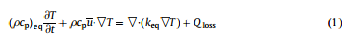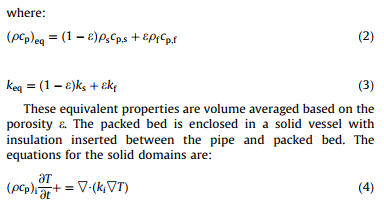Here the subscript i refers to each solid domain (vessel wall, insulation, and tubular insert). Heat losses occur through conduction in the insulation(s) and vessel, and via natural convection at the vessel wall. As will be discussed, temperature-dependent properties of both the fluid and solid phases are used.

## Solver, timestep, and mesh independence

The Navier–Stokes equations are solved with the commercial, finite-volume method, CFD program Star-CCM+1. Due to the simple geometry, an implicit unsteady Reynolds-averaged Navier– Stokes (URANS) formulation is used and the flow is laminar. Convection of heat between the fluid and solid walls is calculated explicitly through an energy equation segregated from the velocity and pressure solution. Conduction through and between the different wall sections is solved with a finite volume stress analysis. A porous region is used to incorporate the one-equation thermal model for the air and a-alumina packed bed, with viscous and inertial losses modifying the velocity and pressure fields as added body forces within the momentum equations. The Navier–Stokes energy equation additionally modifies the fluid density as being proportional to the region porosity. The equations presented here are solved in a 2-D axisymmetric case due to the symmetry of the cylindrical TES vessel.

Model accuracy is a function of both the mesh size and timestep used, with the finite volume formulation being second-order accurate in space and first-order accurate in time. For heat transfer in a straight cylinder the principal source of error will typically arise at the wall, where the wall shear stress (the gradient of the velocity away from the wall) determines the convective heat transfer coefficient. Elsewhere within the pipe, the flow is well behaved and progresses into a fully developed profile. While the implicit solution is unconditionally stable for arbitrary timesteps, the amount of energy transferred between the fluid and solid phases will change and heavily impacts temperature-dependent properties. Mesh and time-step independence for a model is found by systematically decreasing values for each of the mesh size and time step until the solution minimally changes, and can be performed independently of each other. The Courant–Friedrichs– Lewy (CFL) condition provides a guide for choosing a timestep in an implicit solution by evaluating the local velocity and cell size to ensure a particle of fluid does not pass through more than one element (C = u Dt/Dx). This is a minimum requirement for explicit CFD solutions, but can be overly restrictive because as the mesh decreases in size, so too the timestep. Since the implicit solution is stable for all timesteps, this condition can be relaxed to a converged solution instead.

Initially, the domains are set to a constant temperature (e.g., 20 C to correspond to an initial charge, though different temperatures can be applied in each domain). The pressure at the exit is defined and the desired inlet mass flux is set (kg m2 s1 ). A heat transfer coefficient is set at the vessel boundary to estimate the heat losses to the environment (e.g., 3Wm2K1 for all work here). A mesh size of 0.005 m is used, and timesteps of 1.0 and 3.0 s are used for the “short”- and “long”-storage simulations (these details are explained in Section 4). Little variation was seen between the experimental data (whose collection is outlined below) and timesteps of 0.001 (the CFL condition for “short storage”) and 1.0 s, providing a computational time-savings by itself of 1000. A timestep of 15 s deviated from the experimental data and 1.0 s solutions, but was reasonably close for the “short” storage experiment as to provide fast design iterations before a more detailed analysis, if desired.

## Experimental data colletion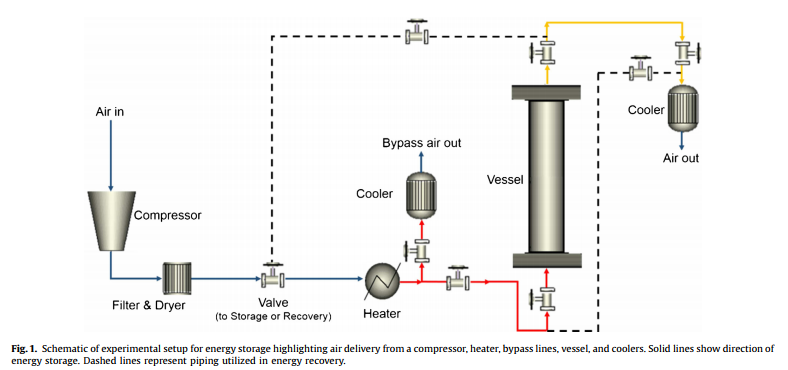These results come from an experimental facility at the City College of New York. A detailed explanation of the setup is available in [13,25]. An overview of that system is provided here, and a schematic is provided in Fig. 1. The a-alumina was purchased commercially (Saint-Gobain Denstone 99; 99% Al2O3). Air is provided by a compressor (Universal Air Products UR57T2- 10E3). The flow path is set by a series of valves, depending on whether heat is being stored or recovered, and the solid lines in Fig. 1 show the direction for storage. The dashed lines are closed during storage but are used during recovery. The air is filtered and dried (nano-Purification Solutions) after the compressor. The air is then heated to a constant inlet temperature (Micropyretics Heaters International MTA925-12). The bypass line lowers the delivered flow rate to the vessel so the supply rate is variable up to 21.7 SCFM. Once the flow rate is set, hot air enters the storage vessel to simulate hot gas from a CSP plant. Air exiting the vessel is cooled before being discharged to the room. The valves can subsequently be manipulated to study the effects of holding the bed with no gas flow or recovering the heat with cold air.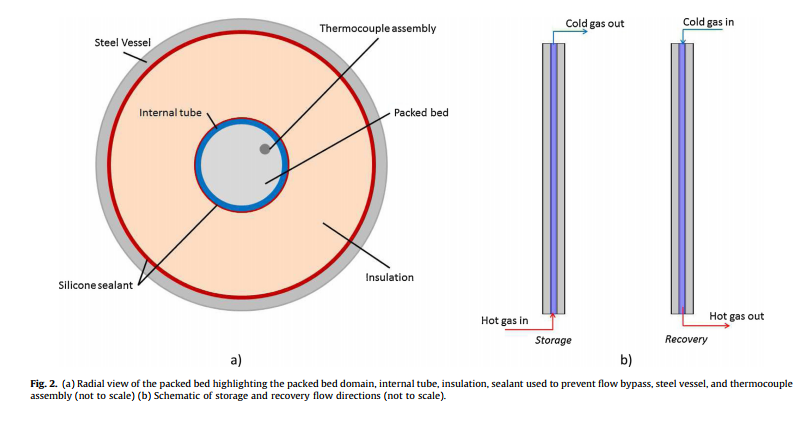The storage vessel domains are shown schematically in Fig. 2, which is the geometry for the experimental data collection/model validation work in this study. The storage vessel is a 10 ft tall steel pressure vessel (1000 NPS schedule 80; O.D. = 10.7500, I.D. = 9.5600). Ten individual one-foot blocks of cylindrical insulation of thickness 3.4700 are located between the packed bed and vessel wall. A ‘tube’ (McMaster Carr #5296K441; O.D. = 2.62500, I.D. = 2.2500) was also inserted between the packed bed and discontinuous insulation to mitigate flow bypassing the packed bed. Sealant at the inlet and outlet sections between the insulation and vessel and the internal tube and insulation further mitigates internal bypass flows. The interior of the tube defines the packed bed diameter, which is 2.2500 (5.72 cm).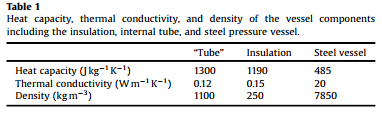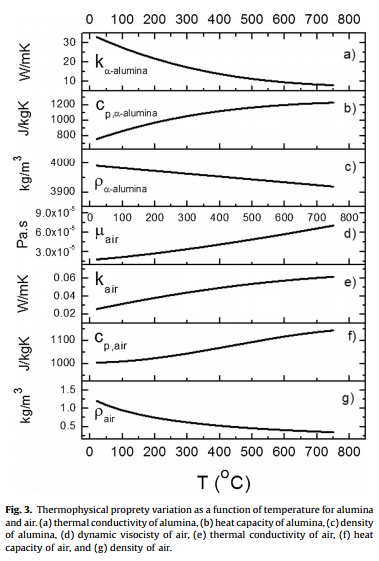The physical properties of the storage container materials are listed in Table 1. The insulation properties are assumed, as only the density was given by the manufacturer. The thermal conductivity and heat capacity are chosen as typical aluminosilicate insulation materials (46% Al2O3, 50% SiO2, 4% Other). The insulation also suffered from cracking, which will have an influence on these parameters, hence it is reasonable to assume typical values. The physical properties of the tube are also assumed for typical rubber values. All of these assumptions are, based on the agreement of modeling with experimental results, reasonable.

The air and alumina properties are modeled here as a function of temperature to be more accurate. For air, the density is calculated from the ideal gas law. The data for the heat capacity and thermal conductivity are from literature , and the dynamic viscosity is given by Sutherland’s formula . For the alumina, the density, heat capacity, and thermal conductivity as a function of temperature are fitted from data . All properties as a function of temperature are shown in Fig. 3.

Polynomial expressions of the data in Fig. 3 are used in the one-equation thermal model. Over the range of temperatures modeled here (20–700 C), the thermal diffusivity (a) of air increases by 700% while the thermal diffusivity of alumina decreases by 84%. This variation with temperature leads to substantive changes in the modeling results, which will be discussed later.

## Alumina results: comparison of model and experiment

Two cases are presented here to highlight the applicability and validity of the one-equation model. The parameters for these two tests are listed in Table 2, with “short storage” corresponding to 20 min of heating at high flow rate and “long storage” corresponding to 3 h of storage at approximately half of the flow rate. The listed temperatures are based on the values recorded during those experimental trials. The porosity was calculated from .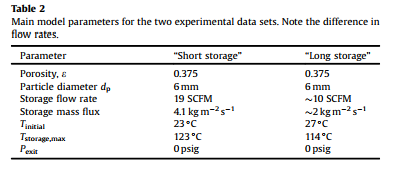The thermal boundary condition at the inlet of the model is a temperature (i.e., Tstorage,max), and specified as a ramp based upon the experimental data in order to approximate the experimental conditions more accurately. The experiment begins with the gas exiting the heaters and then exiting through the bypass. This step allows the heater and air to reach a desired temperature before the valves are manually adjusted to start the heated experiment. When the valve to the vessel is opened and the valve to the bypass closed (t = 0), the peripheral piping system into the vessel also heats, causing the air to lose substantial heat. Thus,this heatloss induces a delay at the boundary that the ramp condition captures satisfactorily without requiring a model of the peripheral piping system. The experimental apparatus does not measure the temperature at the bed inlet; rather the first thermocouple is 0.5 ft into the bed. To estimate a reasonable ramp, the temperature data was estimated at the inlet via extrapolation. The estimated inlet temperatures for the case of long storage at 2 kg m2 s1 are shown in Fig. 4. The dashed-dotted line shows the estimated inlet temperature over time. The dotted line guided this process and was based on extrapolating the data at all of the measured locations back to hbed = 0. The same procedure was used to extrapolate the temperature ramp for the “short storage” case at twice the flow rate.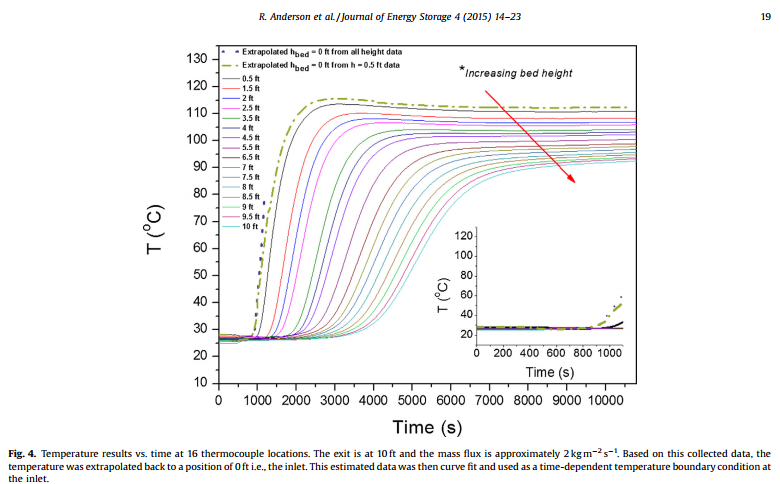The model is first compared to data where there was 20 min of storage at a mass flux of 4.1 kg m2 s 1 , corresponding to 19 SCFM. Though the compressor is rated to 21.7 SCFM, this value tended to drift low and the manual set/observed flow rate is typically closer to 19 SCFM. Without experimental data for the fluctuations in this flow rate, a constant value at 4.1 kg m2 s 1 is reasonable based on experimental observation. The transient results of the storage steps are in Fig. 5, with data presented at five minute (300 s) intervals. Overall, the agreement in temperatures is quite good. The error bars are from three trials and the spread in the data is indicative of minor variations in initial temperature between trials. Considering the estimation of several vessel properties and a slightly variable mass flux seen experimentally, the model agreement with the experimental data is fully acceptable.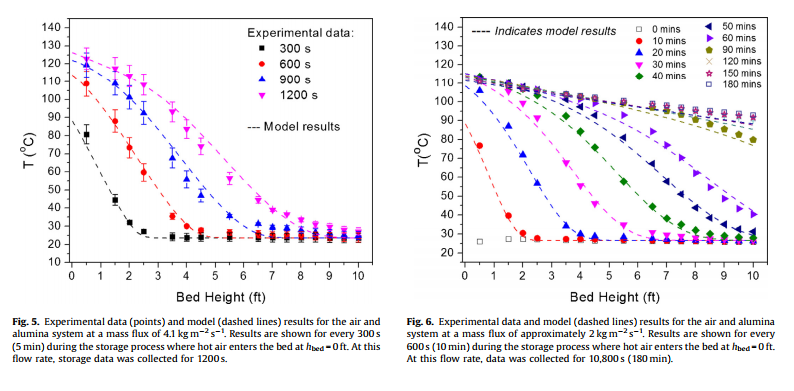Similar agreement between experimental data and numerical modeling results are noted when the flow rate was set at approximately 50% of the original flow rate, i.e., at 2 kg m2 s1 , for the “long storage” experiment. Unlike the first case, the storage test was run for three hours. It was noted experimentally that the flow rate varied over the three hours; initially it was a bit below the set point and then quickly rose above and remained constant. Experimental notes showed flow varying from 1.95 to 2.3 kg m2 s1 , or as stated roughly 2 kg m2 s1 . A time-dependent mass flux, based upon the notes, was used in the model to capture this behavior. Note, however, this flow rate data was collected manually and only at a few times over the three hours, thus it should be considered an additional approximation. An expression was fit to this observed data for implementation in the model. In this experiment, the bed started at a measured 26.7 C, and this temperature was used for the initial condition in all domains. The maximum temperature in these conditions was 114 C. All other material properties (e.g., tube, vessel, insulation, wall heat transfer coefficient) are the same as for the “short storage” experiment. These results are in Fig. 6, and again generally good agreement is obtained. While there are some discrepancies (e.g., model undershoot at long t), this is a reasonable level of accuracy considering the estimates in the model itself (temperature ramp at the inlet, mass flow variation at the inlet) and the estimation of parameters from the experimental setup (vessel properties).

In both flow rate cases, all estimated parameters are reasonable including the material properties, porosity, and boundary condition ramps. One could continue to modify these parameters to ‘optimize’ agreement, but this is not a point of emphasis in this work and would not prove validation. The main goal has been met, showing that the one-equation thermal model approach for a packed bed provides a sufficient level of accuracy for future design studies.

## Effect of temperature-dependent thermophysical properties

Temperature is known to change the thermophysical properties of the system as shown in Fig. 3, and therefore the model results. To explore temperature dependence, the model results are calculated based on thermophysical properties calculated at (1) the average temperature between the high and low temperature, (2) the highest temperature, (3) the lowest temperature, and (4) under variable temperature conditions (i.e., from Fig. 3). Two cases are considered: one for the experimental results presented here where the range of operating temperatures is relatively narrow (20–120 C), and a second where the range is more applicable to a true CSP system (20–700 C).

#### 5.1. Model of air and alumina at 120 C

The model results for both the short- and long-storage are shown in Fig. 7. It is interesting that even under this narrow range of operating temperature, the model produces variable results.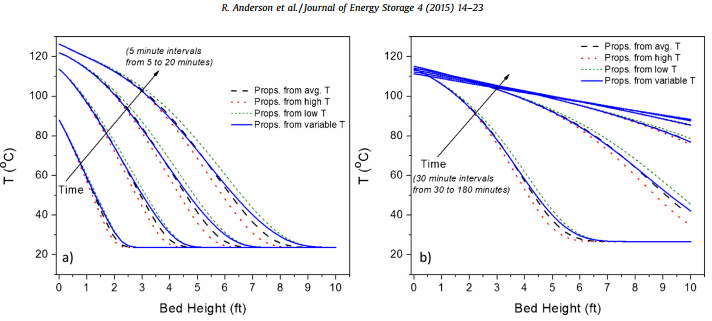Fig. 7. Model results where thermophysical properties are based on the calculated value at an average of the high and low temperature, the high temperature (120 C), the
low temperaure (20 C), or variable (relationships in Fig. 3). (a) Results from “Short” storage of 20 min at a mass flux of 4.1 kg m2 s1
. (b) Results from “Long” storage of
180 min at a mass flux of approximately 2 kg m2 s1

In particular, note that the results based on an average temperature (as might be regularly assumed) and the temperature-dependent case are not the same. The results are indeed close, but still demonstrate a variation, particularly at the downstream edge of the thermal front, which would lead to an over-prediction of system efficiency in both cases. It is visually most noticeable in Fig. 7a at 20 min. While it may be very reasonable to assume a mean temperature for an initial design, those interested in repeated cycles or process control strategies should use temperature dependent parameters for more accurate results. Additionally, it may be difficult to define a true ‘mean’ in variable cycles.

#### 5.2. Model of air and alumina at 700 C

Studies have explored using thermal energy storage (TES) over different temperature ranges [5,39]. For high exergy recovery, it is important to recover heat at the highest temperature possible since the Carnot efficiency is considered as a part of the analysis . To further highlight the effect of temperature-dependent thermophysical-properties, a case is run at 2 kg m2 s1 where the systems starts at 20 C and the inlet is then set at 700 C. For this analysis, no temperature boundary condition ramp is utilized and the model geometry is reused. Only storage results are presented. Again, the results are obtained with properties based on the mean temperature (360 C), the lowest temperature (20 C), the highest temperature (700 C), and with variable properties (20–700 C). These results are shown in Fig. 8.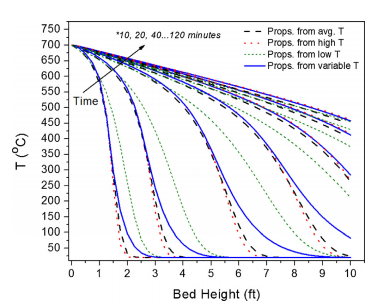Fig. 8. Model results where thermophysical properties are based on the calculated
value at an average of the high and low temperature, the high temperature (700 C),
the low temperaure (20 C), or variable (relationships in Fig. 3). These results
correspond to a mass flux of 2 kg m2 s1 and correspond to 2 h of storage time.

As can be seen, there is substantial change in the thermal front depending on how the thermophysical properties are calculated. In particular, the difference between using an average temperature for the calculation and using the temperature-dependent properties is more substantial at these higher temperatures. Considering the final condition of storage is the initial condition for recovery, the error would compound through cycling. Thus, it is recommended that designers working with materials having large thermophysical property variations over the temperature range of interest implement the property variation in the models.

## Limitations to the one-equation approach

The one-equation thermal model approach is said to work when the thermal conductivity and thermal capacity of the solid are high compared to the heat transfer fluid [12,24]. It is clear from Fig. 3 that these conditions are met in the air/alumina system presented here. In particular the volumetric heat capacity of alumina is 2500–11,500 times higher than air over the temperature range of 20–700 C (at 1 atm). Over the same lowhigh temperature range, the thermal conductivity of the alumina is 1300–140 times higher than the air. Thus, thermal equilibrium is a reasonable assumption in the air/alumina system, meaning the porous media can be reasonably approximated as ‘homogeneous’. This description would be inaccurate for heat transfer fluids with different properties such as a molten salt. As an example, molten salt LiNO3–NaNO3–KNO3–NaNO2 has a heat capacity of about 1.55 J kg1K1 , a density of 1900 kg m3 at 250 C, and a thermal conductivity of 0.55Wm1K1 . A ratio of the alumina volumetric heat capacity to that of this molten salt is only 1.4. Thus, this approach would not be suitable for typical molten salt properties, and a two-equation model with energy balances for both phases would be necessary. Interestingly, for work examining new heat transfer fluids such as supercritical carbon dioxide for high temperature applications (e.g., at 720 C and 275 bar), the one-equation approach may be suitable as the volumetric heat capacity ratio (alumina to sCO2) is 29 and the thermal conductivity ratio is 110.

In situations where thermal equilibrium cannot be assumed, it becomes critically important to (1) use accurate thermophysical properties for Nusselt (Nu), Prandtl (Pr), and Reynolds (Re) in order to calculate the heat transfer coefficient and (2) use a heat transfer coefficient correlation that is closely related to the proposed conditions. The Nusselt number as a function of Pr and Re is a function of the fluid density, viscosity, velocity, heat capacity, thermal conductivity, particle diameter, and bed geometry, and thus thermophysical property variation with temperature can have a noticeable effect. It is often difficult to find an accurate empirical expression for the heat transfer coefficient. Though a range of Nusselt numbers and friction factors have been developed for packed bed systems [41–43], the number of choices that are applicable for a single operating condition result in a range of heat transfer coefficient calculations that therefore lead to multiple exergy solutions and add uncertainty [44,45]. Significant scatter in experimental heat transfer coefficients exists over a range of flow rates [44,45]. Atlow Reynolds numbers, which could be used in TES to minimize pressure drop, older data shows a scatter of two orders of magnitude in determining the fluid/solid heat transfer coeffi- cient . A wide range of additional correlations are provided in [26,43,44,46–49].

Another important consideration in the present work is the Biot number, where Bi < 0.1 allows one to safely neglect intraparticle temperature gradients in the modeling. The results presented here are based on the lowest mass flux of 2 kg m2 s 1 and the vessel dimensions presented in Section 3 of this work. At fixed flow rate, the Biot number steadily climbs with temperature. The variations of the heat transfer coefficient and alumina’s thermal conductivity with temperature are shown in Fig. 9a. The heattransfer coefficient is estimated as in [13,43] and is a function of Re and Pr. The controlling parameter in this case is the thermal conductivity of the alumina. The change in the heat transfer coefficient is only approximately 10% over the range of temperatures shown here, while the thermal conductivity decreased by over 70%. These are shown by the ratios in Fig. 9b (ratio of increasing temperature to initial lower temperature).

Again considering the vessel geometry presented in the experimental section, one can see the combined effects of temperature and mass flux. Presented are low temperature operation (such as the experimental data here at 120 C) and then high temperature operation (an inlet temperature of 700 C). These results are shown in Fig. 10. It becomes clear that at higher temperatures and higher mass fluxes, neglecting intra-particle conduction may no longer be valid. This effect can be incorporated into modeling efforts , though these temperature gradients should be avoided in practical operation for high efficiency TES operations .

## Conclusions

This paper examined a one-equation approach to thermal energy storage in a packed bed of alumina with air as heat transfer fluid. This modeling approach assumes thermal equilibrium between the fluid and solid phases, which is valid here based on the high heat capacity and thermal conductivity of the solid compared to the fluid. The model solves the axial and radial temperature profiles in the packed bed, insulation, and vessel. The model matches experimental data well for two flow-rate conditions. For accuracy, temperature-dependent thermophysical properties of the air and alumina must be used. This effect is particularly important at the high temperatures expected in applications like CSP (e.g., 700 C). Those using this approach should be careful to ensure that thermal equilibrium is valid and the Biot number remains less than 0.1 to avoid intra-particle conduction effects.

### Authors:

Ryan Andersona,e, *, Liana Batesa , Erick Johnsonb,e , Jeffrey F. Morrisc,d aDepartment of Chemical and Biological Engineering, Montana State University, Bozeman, MT 59717, United States bDepartment of Mechanical & Industrial Engineering, Montana State University, Bozeman, MT 59717, United States c Department of Chemical Engineering, City College of New York, New York, NY 10031, United States d Levich Institute, City College of New York, New York, NY 10031, United States eEnergy Research Institute, Montana State University, Bozeman, MT 59717, United States

This is an open access article under the CC BY license

References  H.L. Zhang, J. Baeyens, J. Degrève, G. Cacères, Concentrated solar power plants: review and design methodology, Renew. Sustain. Energy Rev. 22 (2013) 466– 481.  U.S. Department of Energy, Energy Efficiency & Renewable Energy. http:// energy.gov/sites/prod/files/2013/11/f4/55296.pdf, 2012 (accessed 7.10.14).  P. Li, Energy storage is the core of renewable energy technologies, IEEE Nanotechnol. Mag. 4 (2008) 3–18.  International Renewable Energy Agency, Renewable Energy Technologies: Cost Analysis Series, Volume 1: Power Sector Issue 2/5, Concentrating Solar Power, June 2012.  S. Kuravi, J. Trahan, D.Y. Goswami, M.M. Rahman, E.K. Stefanakos, Thermal energy storage technologies and systems for concentrating solar power plants, Prog. Energy Combust. Sci. 39 (4) (2013) 285–319.  US. Department of Energy, SunShot vision study. Chap. 5. Feb. 2012, p. 115.  P. Li, J. Van Lew, C. Chan, W. Karaki, J. Stephens, J.E. O’Brien, Similarity and generalized analysis of efficiencies of thermal energy storage systems, Renew. Energy 39 (1) (2012) 388–402.  E. Oró, A. de Gracia, A. Castell, M.M. Farid, L.F. Cabeza, Review on phase change materials (PCMs) for cold thermal energy storage applications, Appl. Energy 99 (2012) 513–533.  B. Zalba, J.M. Marı’n, L.F. Cabeza, H. Mehling, Review on thermal energy storage with phase change: materials, heat transfer analysis and applications, Appl. Therm. Eng. 23 (3) (2003) 251–283.  J.P. Coutier, E.A. Farber, Two applications of a numerical approach of heat transfer process within rock beds, Solar Energy 29 (6) (1982) 451–462.  G. Zanganeh, A. Pedretti, S. Zavattoni, M. Barbato, A. Steinfeld, Packed-bed thermal storage for concentrated solar power—pilot-scale demonstration and industrial-scale design, Solar Energy 86 (10) (2012) 3084–3098.  K.A.R. Ismail, R. Stuginsky Jr, A parametric study on possible fixed bed models for PCM and sensible heat storage, Appl. Therm. Eng. 19 (7) (1999) 757–788.  R. Anderson, S. Shiri, H. Bindra, J.F. Morris, Experimental results and modeling of energy storage and recovery in a packed bed of alumina particles, Appl. Energy 119 (2014) 521–529.  A.M. Waked, Solar energy storage in rocks, Solar Wind Technol. 3 (1) (1986) 27–31.  H. Bindra, P. Bueno, J.F. Morris, R. Shinnar, Thermal analysis and exergy evaluation of packed bed thermal storage systems, Appl. Therm. Eng. 52 (2) (2013) 255–263.  D. Laing, W.D. Steinmann, R. Tamme, C. Richter, Solid media thermal storage for parabolic trough power plants, Solar Energy 80 (10) (2006) 1283–1289.  S.L. Aly, A.I. El-Sharkawy, Effect of storage medium on thermal properties of packed beds, Heat Recovery Syst. CHP 10 (5) (1990) 509–517.  K.G. Allen, T.W. von Backström, D.G. Kröger, Packed rock bed thermal storage in power plants: design considerations, Energy Procedia 49 (2014) 666–675.  M.A. Al-Nimr, M.K. Abu-Qudais, M.D. Mashaqi, Dynamic behaviour of a packed bed energy storage system, Energy Convers. Manage. 37 (1) (1996) 23–30.  A.E. Saez, B.J. McCoy, Dynamic response of a packed bed thermal storage system—a model for solar air heating, Solar Energy 29 (3) (1982) 201–206.  M.Y. Haller, C.A. Cruickshank, W. Streicher, S.J. Harrison, E. Andersen, S. Furbo, Methods to determine stratification efficiency of thermal energy storage processes—review and theoretical comparison, Solar Energy 83 (10) (2009) 1847–1860.  H. Bindra, P. Bueno, J.F. Morris, Sliding flow method for exergetically efficient packed bed thermal storage, Appl. Therm. Eng. 64 (1) (2014) 201–208.  T.E. Schumann, Heat transfer: a liquid flowing through a porous prism, J. Franklin Inst. 208 (3) (1929) 405–416.  D. Vortmeyer, R.J. Schaefer, Equivalence of one-and two-phase models for heat transfer processes in packed beds: one dimensional theory, Chem. Eng. Sci. 29 (2) (1974) 485–491.  S. Shiri, A model of transient heat transfer in a packed bed of alumina particles, MS Thesis, City University of New York, 2013.  D.A. Nield, A. Bejan, Convection in Porous Media, Springer, 2006.  H. Singh, R.P. Saini, J.S. Saini, A review on packed bed solar energy storage systems, Renew. Sustain. Energy Rev. 14 (3) (2010) 1059–1069.  H. Bindra, P. Bueno, Optimum process design of packed bed type thermal storage systems and other applications, WO patent 2013015834.  A.A. Adeyanju, K. Manohar, Theoretical and experimental investigation of heat transfer in packed beds, Res. J. Appl. Sci. 4 (5) (2009) 166–177.  Z. Guo, T.S. Zhao, Lattice Boltzmann model for incompressible flows through porous media, Phys. Rev. E 66 (3) (2002) 036304.  P. Nithiarasu, K.N. Seetharamu, T. Sundararajan, Natural convective heat transfer in a fluid saturated variable porosity medium, Int. J. Heat Mass Transfer 40 (16) (1997) 3955–3967.  CD-Adapco User Guide. STAR-CCM+ Version (10.02.012), 2015.  S. Ergun, Fluid flow through packed columns, Chem. Eng. Prog. 48 (2) (1952) 89–94.  D.A. Nield, A. Bejan, Convection in Porous Media, Springer-Verlag, 1992.  K. Kadoya, N. Matsunaga, A. Nagashima, Viscosity and thermal conductivity of dry air in the gaseous phase, J. Phys. Chem. Ref. Data 14 (4) (1985) 947–970.  F.M. White, Viscous Fluid Flow, 3rd ed., McGraw Hill, New York, 2006.  M. Munro, Evaluated material properties for a sintered alpha-alumina, J. Am. Ceram. Soc. 80 (8) (1997) 1919–1928.  F. Benyahia, K.E. O’Neill, Enhanced voidage correlations for packed beds of various particle shapes and sizes, Part. Sci. Technol. 23 (2) (2005) 169–177.  U. Herrmann, D.W. Kearney, Survey of thermal energy storage for parabolic trough power plants, J. Solar Energy Eng. 124 (2) (2002) 145–152.  Novel Molten Salts Thermal Energy Storage for Concentrating Solar Power Generation http://www1.eere.energy.gov/solar/pdfs/ csp_pr2011_university_of_alabama.pdf.  R. Singh, R.P. Saini, J.S. Saini, Nusselt number and friction factor correlations for packed bed solar energy storage system having large sized elements of different shapes, Solar Energy 80 (7) (2006) 760–771.  H. Singh, R.P. Saini, J.S. Saini, Performance of a packed bed solar energy storage system having large sized elements with low void fraction, Solar Energy 87 (2013) 22–34. Fig. 10. Biot number as a function for mass flux for temperatures of 120 C and 700 C. These results illustrate that for certain combinations of temperature and flow, it may be inappropriate (Bi > 0.1) to neglect intraparticle conduction. 22 R. Anderson et al. / Journal of Energy Storage 4 (2015) 14–23  G. Nellis, S. Klein, Heat Transfer, Cambridge University Press, New York, 2009.  E. Achenbach, Heat and flow characteristics of packed beds, Exp. Therm. Fluid Sci. 10 (1) (1995) 17–27.  P.J. Heggs, D. Burns, Single-blow experimental prediction of heat transfer coefficients: a comparison of four commonly used techniques, Exp. Therm. Fluid Sci. 1 (3) (1988) 243–251.  A.G. Dixon, D.L. Cresswell, Theoretical prediction of effective heat transfer parameters in packed beds, AIChE J. 25 (4) (1979) 663–676.  W.L. McCabe, J.C. Smith, P. Harriott, Unit Operations of Chemical Engineering, 7th ed., McGraw-Hill, New York, 2015.  J. Pallares, F.X. Grau, A modification of a Nusselt number correlation for forced convection in porous media, Int. Commun. Heat Mass Transfer 37 (9) (2010) 1187–1190.  N. Wakao, S. Kaguei, T. Funazkri, Effect of fluid dispersion coefficients on particle-to-fluid heat transfer coefficients in packed beds: corr

We will be happy to hear your thoughts